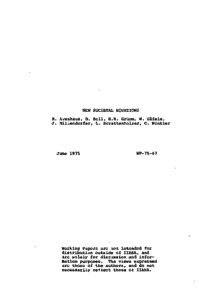# New Societal Equations

Häfele, W., Avenhaus, R., Bell, D.E., Gruemm, H.-R., Winkler, C., & Schrattenholzer, L. (1975). New Societal Equations. IIASA Working Paper. IIASA, Laxenburg, Austria: WP-75-067Preview Text WP-75-067.pdf Download (832kB) | Preview

## Abstract

In a recent paper, W. Haefele established a number of phenomenological equations describing the behavior of a model society. The state variables of this model society were gross national product, population, energy consumption and risk acceptance.

In this paper, the state of the discussion within the IIASA energy project at the time being shall be fixed. Several new sets of equations will be established which extend the set given by Haefele and Manne in the following sense: capital will be included as another state variable; a finite asymptotic population will be assumed; there are several primary energy sources (fossil and nuclear).

We will outline three different approaches, namely: (1) an approach where a complete system of equations, including one primary energy source, is established and where the topological features (separatrices, fix points, etc.) can be studied in detail; (2) a "control theoretical approach," including two primary energy sources, where we limit the number of state variables in such a way that there remains only one "control variable" subject to optimization with respect to an appropriate objective function; and (3) a "linear programming approach" where we introduce the same number of energy supply variables as in the work of Haefele and Manne, and where we optimize the (more than one) free state variables according to different objective functions. The total energy demand is either taken from a model of the first kind or is assumed to be an independent control variable subject to optimization.

Item Type: Monograph (IIASA Working Paper) Energy Program (ENP) IIASA Import 15 Jan 2016 01:42 27 Aug 2021 17:07 https://pure.iiasa.ac.at/361View Item